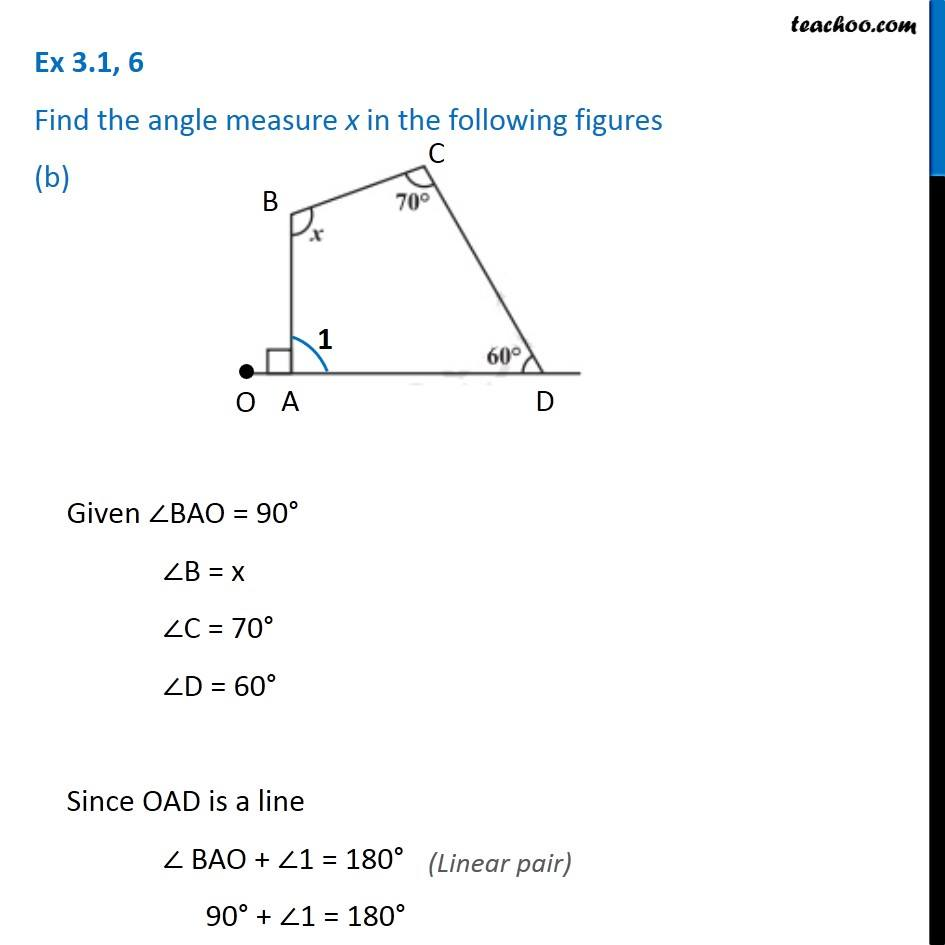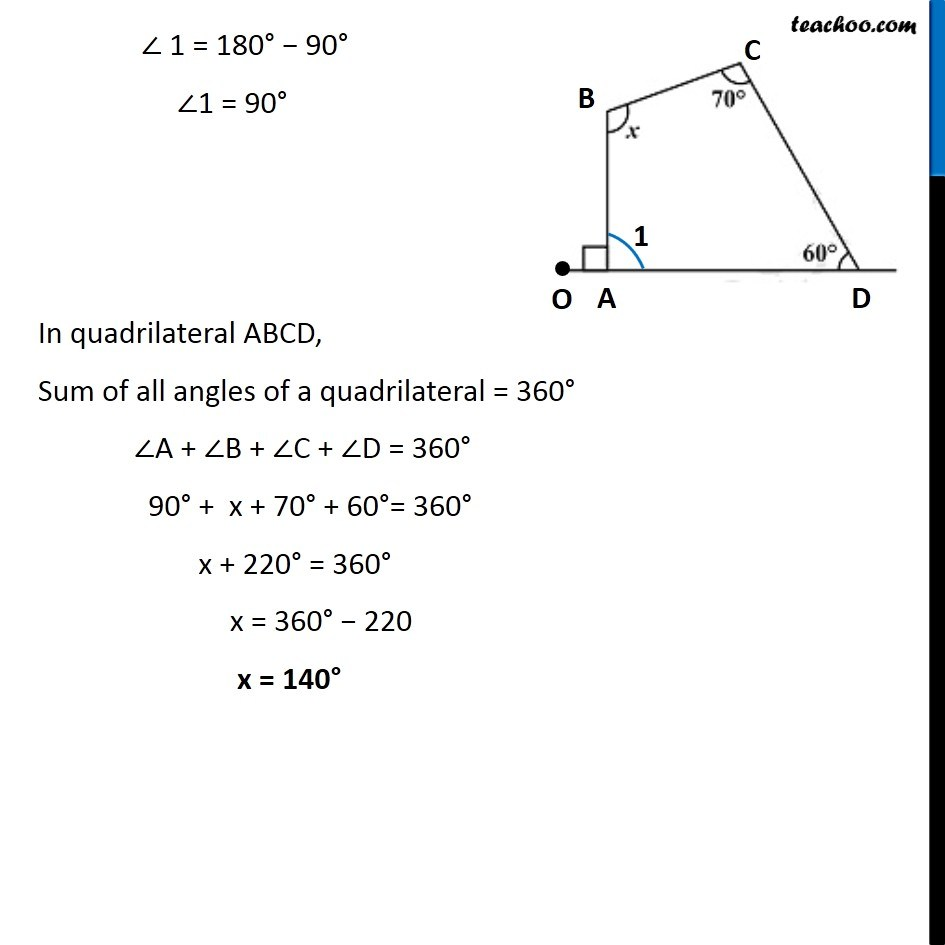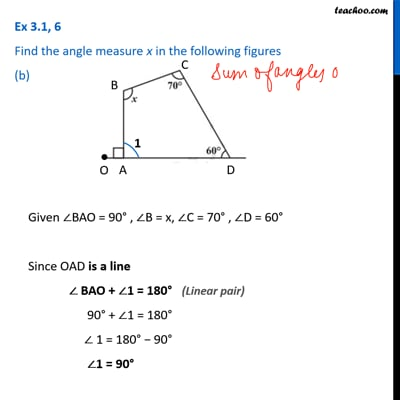Ex 3.1

Chapter 3 Class 8 Understanding Quadrilaterals
Serial order wiseThis video is only available for Teachoo black users

### Transcript

Ex 3.1, 6 Find the angle measure x in the following figures (b) Given ∠BAO = 90° ∠B = x ∠C = 70° ∠D = 60° Since OAD is a line ∠ BAO + ∠1 = 180° 90° + ∠1 = 180° ∠ 1 = 180° − 90° ∠1 = 90° In quadrilateral ABCD, Sum of all angles of a quadrilateral = 360° ∠A + ∠B + ∠C + ∠D = 360° 90° + x + 70° + 60°= 360° x + 220° = 360° x = 360° − 220 x = 140°